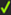﻿ MechDesigner Reference & User Interface > Dialog-Boxes > Dialog: Function-Block: Point-Data

# Dialog: Function-Block: Point-Data

Navigation:  MechDesigner Reference & User Interface > Dialog-Boxes >

# Dialog: Function-Block: Point-Data

## Point-Data FB

Add a Point-Data FB to measure the motion of a Point, with respect to the Base-Part.

Its three output-connectors give, with respect to the the Point's:

motion-values parallel to the X-axis

motion-values parallel to the Y-axis

Magnitude of the motion-values equal to:

o√(X2 + Y2)

Note: Motion-Values include the Position, Velocity, and Acceleration of the Point.

### Open Point-Data FB dialog-boxEdit the Point-Data FB:

 1.Double-click the Point-Data FB in the graphic-area - or - 1.

The Point-Data dialog-box is now open.

### Point-Data dialog-boxSelect a Point:

 1.a. Click a Point in the graphic-area

To change the Point is in the selection-box, then:

 1.b. Click a different Point in the graphic-area

When the Point is in the selection-box.

 2.Clickto close the Point-Data dialog-box### To use the Point-Data FB

How to Use :

Connect a wire from its output-connector to the input-connector of a Graph FB - plot the Position, Velocity and Acceleration of the Point in the X and Y–axis directions - see also: Top-Tip

Connect a wire from its output-connector to the input-connector of a Motion FB - use the motion of the Point as the independent (X-axis) for a Motion.

Top-Tip :

To plot the three motion-derivatives of the same motion-value e.g. Position, Velocity, and Acceleration in one Graph

1.Drag three times from one output-connector to three different input-connectors of a Graph FB

2.Use the Y–axis display options in the Graph dialog-box to select each motion-derivatives.